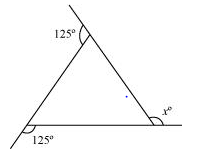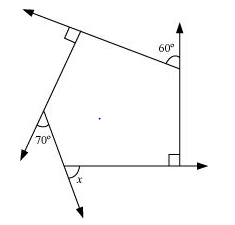# NCERT Solutions for Class 8 Maths Chapter 3 Exercise 3.2

In this page we have NCERT Solutions for Class 8 Maths Chapter 3 Exercise 3.2.This exercise has questions about Sum of the Measures of the Exterior Angles of a Polygon, how to find the xterior angles given inetrior angles. Hope you like them and do not forget to like , social share and comment at the end of the page.

## NCERT Solutions for Class 8 Maths Chapter 3 Exercise 3.2

Question 1
Find x in the following figures
a.b.We know that the sum of all exterior angles of any polygon is 360º.
a. 125° + 125° +x= 360°
250° +x= 360°
x= 110°
b.
60° + 90° + 70° +x+ 90° = 360°
310° +x= 360°
x= 50°

Question 2
Find the measure of each exterior angle of a regular polygon of
(i) 9 sides
(ii) 15 sides
(i) Sum of all exterior angles of the given polygon = 360º
Each exterior angle of a regular polygon has the same measure.
Thus, measure of each exterior angle of a regular polygon of 9 sides
=360/9= 40°
(ii) Sum of all exterior angles of the given polygon = 360º
Each exterior angle of a regular polygon has the same measure.
Thus, measure of each exterior angle of a regular polygon of 15 sides
=360/15=24°

Question 3
How many sides does a regular polygon have if the measure of an exterior angle is 24°?
Sum of all exterior angles of the given polygon = 360º
Measure of each exterior angle = 24º
Thus, number of sides of the regular polygon
=360/24=15

Question 4
How many sides does a regular polygon have if each of its interior angles is 165°?
Answer -Measure of each interior angle = 165°
Measure of each exterior angle = 180° − 165° = 15°
The sum of all exterior angles of any polygon is 360º.
Thus, number of sides of the polygon
=360/15=24°

Question 5
(a) Is it possible to have a regular polygon with measure of each exterior angle as 22°?
(b) Can it be an interior angle of a regular polygon? Why?
The sum of all exterior angles of all polygons is 360º. Also, in a regular polygon, each exterior angle is of the same measure. Hence, if 360º is a perfect multiple of the given exterior angle, then the given polygon will be possible.
(a) Exterior angle = 22°
360º is not a perfect multiple of 22º. Hence, such polygon is not possible.
(b) Interior angle = 22°
Exterior angle = 180° − 22° = 158°
Such a polygon is not possible as 360° is not a perfect multiple of 158°.

Question 6
(a) What is the minimum interior angle possible for a regular polygon?
(b) What is the maximum exterior angel possible for a regular polygon?
Consider a regular polygon having the lowest possible number of sides (i.e., an equilateral triangle). The exterior angle of this triangle will be the maximum exterior angle possible for any regular polygon.
Exterior angle of an equilateral triangle = 360/3=120
Hence, maximum possible measure of exterior angle for any polygon is 120º. Also, we know that an exterior angle and an interior angle are always in a linear pair.
Hence, minimum interior angle = 180º − 120° = 60º

## Summary

1. NCERT Solutions for Class 8 Maths Chapter 3 Exercise 3.2 has been prepared by Expert with utmost care. If you find any mistake.Please do provide feedback on mail. You can download the solutions as PDF in the below Link also
2. This chapter 3 has total 4 Exercise 3.1 ,3.2,3.3 and 3.4. This is the Second exercise in the chapter.You can explore previous exercise of this chapter by clicking the link below